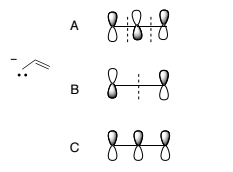# Problem: Consider the Molecular Orbitals (MO’s) of the allyl anion. Which are the HOMO and LUMO?  1) HOMO = B, LUMO = C 2) HOMO = B, LUMO = A 3) HOMO = C, LUMO = A 4) HOMO = A, LUMO = C 5) HOMO = C, LUMO = B

93% (71 ratings)
###### Problem Details

Consider the Molecular Orbitals (MO’s) of the allyl anion. Which are the HOMO and LUMO?

1) HOMO = B, LUMO = C

2) HOMO = B, LUMO = A

3) HOMO = C, LUMO = A

4) HOMO = A, LUMO = C

5) HOMO = C, LUMO = BWhat scientific concept do you need to know in order to solve this problem?

Our tutors have indicated that to solve this problem you will need to apply the HOMO LUMO concept. You can view video lessons to learn HOMO LUMO. Or if you need more HOMO LUMO practice, you can also practice HOMO LUMO practice problems.

What is the difficulty of this problem?

Our tutors rated the difficulty ofConsider the Molecular Orbitals (MO’s) of the allyl anion. W...as low difficulty.

How long does this problem take to solve?

Our expert Organic tutor, Johnny took 4 minutes and 14 seconds to solve this problem. You can follow their steps in the video explanation above.

What professor is this problem relevant for?

Based on our data, we think this problem is relevant for Professor Ayres' class at UC.